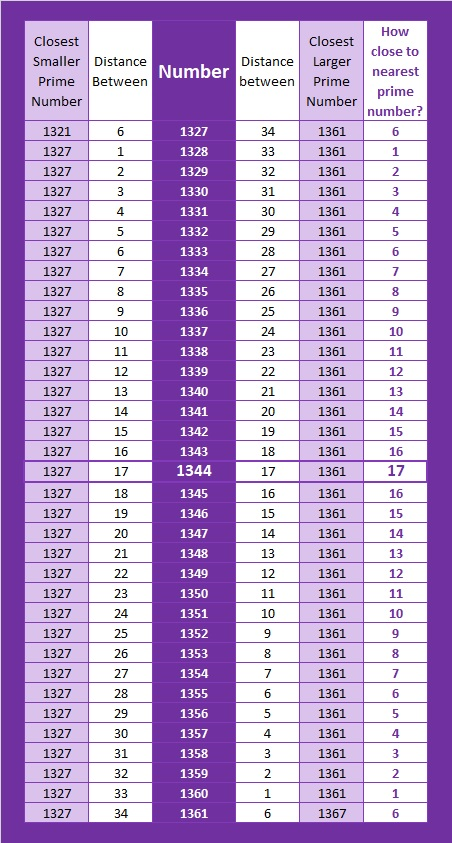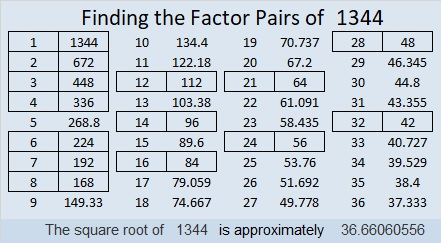# How Far Away Is 1344 from the Nearest Prime Number?

Contents

### The Distance 1344 is from the Nearest Prime Number:

1344 is 17 numbers away from the nearest prime number. 17-away is a new record for distance to the closest prime number!1344 will hold that record until prime number 2179 claims it with being 18-away from the nearest prime:
2161–(18 composite numbers)–2179–(24 composite numbers)–2203

OEIS.org mentioned the previous two numbers, 1342 and 1343, breaking the 15-away and the 16 away records respectively, but didn’t mention this one. I guess it considered 1344 being the order of a perfect group to be more important.

### Factors of 1344:

• 1344 is a composite number.
• Prime factorization: 1344 = 2 × 2 × 2 × 2 × 2 × 2 × 3 × 7, which can be written 1344 = 2⁶ × 3 × 7
• 1344 has at least one exponent greater than 1 in its prime factorization so √1344 can be simplified. Taking the factor pair from the factor pair table below with the largest square number factor, we get √1344 = (√64)(√21) = 8√21
• The exponents in the prime factorization are 6, 1 and 1. Adding one to each exponent and multiplying we get (6 + 1)(1 + 1)(1 + 1) = 7 × 2 × 2 = 28. Therefore 1344 has exactly 28 factors.
• The factors of 1344 are outlined with their factor pairs in the graphic below.### Sum-Difference Puzzles:

84 has six factor pairs. One of those pairs adds up to 25, and another one subtracts to 25. Put the factors in the appropriate boxes in the first puzzle.

1344 has fourteen factor pairs. One of the factor pairs adds up to ­100, and a different one subtracts to 100. If you can identify those factor pairs, then you can solve the second puzzle!The second puzzle is really just the first puzzle in disguise. Why would I say that?

This site uses Akismet to reduce spam. Learn how your comment data is processed.